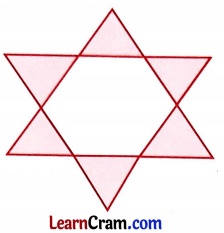# DAV Class 7 Maths Chapter 8 Worksheet 8 Solutions

The DAV Maths Book Class 7 Solutions Pdf and DAV Class 7 Maths Chapter 8 Worksheet 8 Solutions of Triangle and Its Properties offer comprehensive answers to textbook questions.

## DAV Class 7 Maths Ch 8 WS 8 Solutions

Question 1.
Write whether the following statements are True or False. Correct the false statements to make them true.
(i) The angle bisectors of a triangle meet at its circumcentre.
False.
Correct Statement: The angle bisectors of a triangle meet at its incentre.

(ii) The incentre of a triangle is equidistant from its sides.
True.

(iii) The incircle of a triangle passes through its vertices.
False.
Correct Statement: The incentre of a triangle touches its sides.

(iv) The incentre of a triangle always lies in the exterior of the triangle.
False.
Correct Statement: The incentre of a triangle always lie in the interior of the triangle.

## DAV Class 7 Maths Chapter 8 Value Based Questions

Question 1.
Due to storm and rain a tree broke and fell on a road which prevented the people and vehicles to pass by. A group of children decided to solve the problem. They lifted the broken part and put it aside. All were very thankful to these brave children.(i) If the width of the road was 4m and length of the broken part removed was 5m, what was the height of the tree?
(ii) What value of the children is depicted here?
(i) Let the height of the remaining tree portion of tree be hm.
Width of the road, BC = 4m [Given]
Length of the broken part, AC = 5m [Given]
Now, in right angled ΔABC,
AB2 + BC2 = AC2 [By Pythagoras theorem]
⇒ (h)2 + (4)2 = (5)2
⇒ h2 +16 = 25 Tree
⇒ h2 = 25 – 16
⇒ h2 = 9 w
⇒ h = 3m
Hence, the height of the tree was (h + 5) m i.e., (3 + 5) m = 8 m.

(ii) Value: Children have helpful nature.

Question 2.
For a Rangoli Competition the four participants of the Indira House drew the following Rangoli. They drew a regular hexagon of side 8 cm and then drew 6 equilateral triangles of same size.(i) What is the length of each side of the equilateral triangle?
(ii) What value of the children is depicted here?